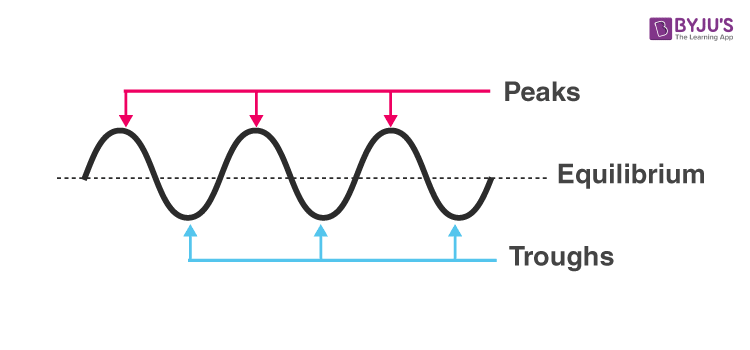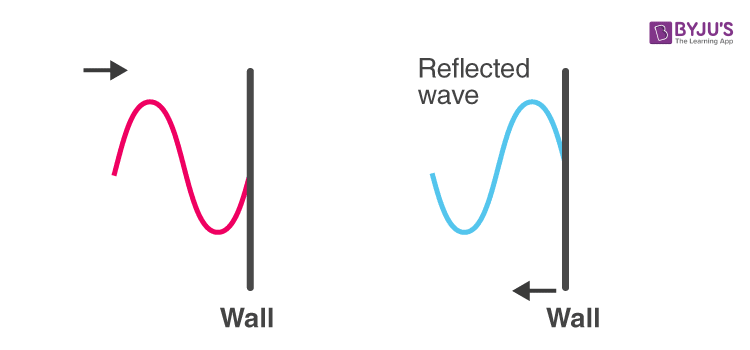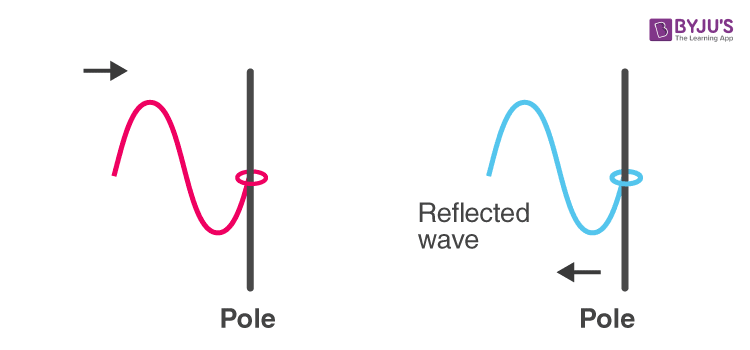# Transverse Waves

Waves are everywhere, and we can describe waves as disturbances that travel through a medium from one location to another. Waves come in many shapes and forms. While all waves share the same basic characteristic properties and behavior, some waves can be distinguished from other waves based on some observable characteristics. One of the ways to categorize is on the basis of the direction of movement of the individual particles of the medium relative to the direction of the wave propagation. Categorizing waves on this basis leads to two notable categories: transverse waves and longitudinal waves. A transverse wave is a wave in which particles move perpendicular to the direction of the wave propagation. A longitudinal wave is a wave in which the particles move in a direction parallel to the wave propagation. In this article, our focus will be on transverse waves and their characteristics. You can check out our article on longitudinal waves to understand their characteristics.

## What is a Transverse Wave?

In Physics, a transverse wave is a moving wave whose oscillations are perpendicular to the direction of the wave. A simple demonstration of the wave can be created on a horizontal length of the string by securing one end of the string and moving the other up and down. Light is another example of a transverse wave, where the oscillations are electric and magnetic fields that are at right angles to the ideal light rays that describe the direction of propagation.

Transverse waves commonly occur in elastic solids, oscillations, in this case, are the displacement of solid particles from their relaxed position, in the direction perpendicular to the propagation of the wave. Since these displacements correspond to local shear deformation of the material, the transverse waves of this form are known as a shear wave. In seismology, shear waves are also known as secondary waves or S-waves.

## Examples of Transverse waves

Some examples of transverse waves are listed below:

• The ripples on the surface of the water
• Electromagnetic waves
• Stadium or a human wave
• Ocean Waves
• The secondary waves of an earthquake

## Speed of a Transverse Wave

Transverse waves are composed of peaks and troughs.  The peak is the top point of the wave and trough is the bottom point of the wave. Refer to the diagram below for the visual representation of these terms.Following are a few important terminologies to know:

• Amplitude – The maximum displacement of a particle from its equilibrium position.
• Wavelength – The distance from either one peak to the next peak, or one trough to the next trough.
• Period – It is the time it takes for two successive peaks to pass through a fixed point.
• Frequency – The number of wavelengths that pass through a given point in a second.

### How do we calculate the speed of a wave on a string?

The velocity of a wave is calculated by dividing the distance traveled by the time it took to travel that distance. For waves, this is calculated by dividing the wavelength by the period as follows:

$$\begin{array}{l}v=\frac{\lambda }{T}\end{array}$$

We can take the inverse proportionality to period and frequency and apply it to this situation as follows:

$$\begin{array}{l}v=\frac{\lambda }{T}\end{array}$$
$$\begin{array}{l}v=\lambda \frac{1}{T}\end{array}$$
$$\begin{array}{l}v=\lambda\,f\end{array}$$

### Speed of a Wave on a Vibrating String

Musical instruments such as piano and guitars use vibrating strings to produce music. The speed of a wave on this kind of string is proportional to the square root of the tension in the string and inversely proportional to the square root of the linear density of the string. This can be formulated as follows:

$$\begin{array}{l}v=\sqrt{\frac{T}{\mu}}\end{array}$$

## Reflection of Transverse Waves

The way in which a transverse wave reflects depends on whether it is fixed at both ends. First, let us discuss a case where the waves are fixed at both ends.

The image below shows a transverse wave that is reflected from a fixed end. When a transverse wave meets a fixed end, the wave is reflected but inverted. This swaps the peak with troughs and the troughs with peaks.The image below shows a transverse wave on a string that meets a free end. The wave is reflected, but unlike a transverse wave with a fixed end, it is not inverted.Stay tuned to BYJU’S and FALL IN LOVE WITH LEARNING!

Since you are here, you might be interested in the following articles:

Test your Knowledge on Transverse Waves